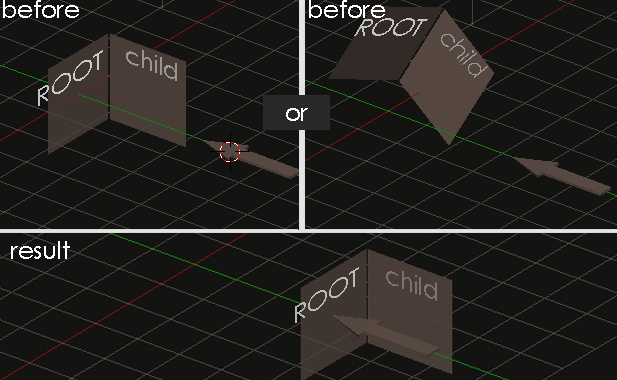# python: How calculate Matrix translate/Rotate one align an object with other ?

Guy. I’m trying to learn a bout Matrix using python script.
I have a problem that troubled me for three days,And my results have been wrong…

On my scene : There is a “Arrow” for leading a group of obects, 2 X Plane ,one as a “Root” , another is “Child” of the Root ,all of them already have their own position.Now,I translate and rotate the “Arrow” manually,and run my script tring realize below with Matrix :
The “Root” match the position of the “Arrow”, according to the matrix of the “Arrow”.The “Child” then produces a displacement, according to the original relationship of the “Root”

``````
# encoding: UTF-8
import bpy
import mathutils
import math
from mathutils import Matrix

# root
root = bpy.data.objects['Plane']
root_mat =  root.matrix_world
root_loc,root_rot,root_scale = root_mat.decompose()
root_loc_mat= Matrix.Translation(root_loc)
root_rot_mat= root_rot.to_matrix().to_4x4()

# target "Arrow"
target = bpy.data.objects['Arrow']
target_mat = target.matrix_world
target_loc,target_rot,target_scale = target_mat.decompose()
target_loc_mat= Matrix.Translation(target_loc)
target_rot_mat= target_rot.to_matrix().to_4x4()

# child
child = bpy.data.objects['Plane.001']
child_mat = child.matrix_world
child_loc,child_rot,child_scale = child_mat.decompose()
child_loc_mat= Matrix.Translation(child_loc)
child_rot_mat= child_rot.to_matrix().to_4x4()

# root - target , Calculate their differences
rt_loc =  target_loc - root_loc
rt_rot =root_rot.rotation_difference(target_rot)
rt_loc_mat = Matrix.Translation(rt_loc)
rt_rot_mat = rt_rot.to_matrix().to_4x4()

# child - root , Calculate their differences
cr_loc = child_loc - root_loc
cr_rot = child_rot.rotation_difference(root_rot)
cr_loc_mat = Matrix.Translation(cr_loc)
cr_rot_mat = cr_rot.to_matrix().to_4x4()

# Test
root.matrix_world  = root_loc_mat * rt_loc_mat * rt_rot_mat * root_rot_mat
child.matrix_world = root.matrix_world * cr_loc_mat * cr_rot_mat

``````

Please forgive my poor English , hope to get help !!